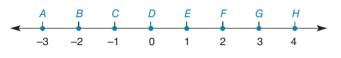Chapter 1.3, Problem 27E### Elementary Geometry for College St...

6th Edition
Daniel C. Alexander + 1 other
ISBN: 9781285195698

#### Solutions

Chapter
Section### Elementary Geometry for College St...

6th Edition
Daniel C. Alexander + 1 other
ISBN: 9781285195698
Textbook Problem
1 views

# In Exercises 9 to 28, use the drawings as needed to answer the following questions.Using the number line provided, name the point thata) is the midpoint of A E ¯ .b) is the endpoint of a segment of length 4 , if the other endpoint is point G.c) has a distance from B equal to 3 ( A C ) .Exercises 27, 28

To determine

a)

To find:

The point that is the midpoint of AE¯.

Explanation

Given:

The given figure is,

Approach:

Consider the given figure,

Figure (1)

Since length of AE¯ is 4 units

To determine

b)

To find:

The point that is the endpoint of a segment of length 4, if the other endpoint is point G.

To determine

c)

To find:

The point that has a distance from B equal to 3(AC).

### Still sussing out bartleby?

Check out a sample textbook solution.

See a sample solution

#### The Solution to Your Study Problems

Bartleby provides explanations to thousands of textbook problems written by our experts, many with advanced degrees!

Get Started

#### Find f. f(x) = 2x + 3ex

Calculus: Early Transcendentals

#### Evaluate the expression sin Exercises 116. (2)3

Finite Mathematics and Applied Calculus (MindTap Course List)

#### In Problems 7-34, perform the indicated operations and simplify. 28.

Mathematical Applications for the Management, Life, and Social Sciences

#### Evaluate the integral. 2. 12x(x+1)2dx

Single Variable Calculus: Early Transcendentals